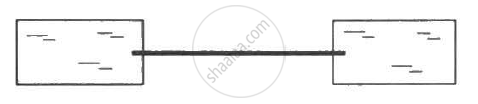Share

# Following Figure Shows Two Adiabatic Vessels, Each Containing a Mass M of Water at Different Temperatures. the Ends of a Metal Rod of Length L, Area of Cross Section a and Thermal Conductivity - Physics

ConceptHeat Transfer Conduction

#### Question

Following figure  shows two adiabatic vessels, each containing a mass m of water at different temperatures. The ends of a metal rod of length L, area of cross section A and thermal conductivity K, are inserted in the water as shown in the figure. Find the time taken for the difference between the temperatures in the vessels to become half of the original value. The specific heat capacity of water is s. Neglect the heat capacity of the rod and the container and any loss of heat to the atmosphere.#### Solution

Rate of transfer of heat from the rod is given as
(DeltaQ)/(Deltat) = (KA(T_1 - T_2))/l

In time t, the temperature difference becomes half.

In time Δt, the heat transfer from the rod will be given by

DeltaQ = (KA(T_1 - T_2))/l Deltat............(i)

Heat loss by water at temperature T1 is equal to the heat gain by water at temperatureT2.

Therefore, heat loss by water at temperature T1 in time Deltat is  given by

DeltaQ=ms(T_1 - T_1) ............(ii)

From equation (i) and (ii),

ms ( T_1 - T_1' ) = (KA(T_1 - T_2))/l Delta t

⇒ T_1' = T_1 - (KA(T_1 - T_2))/(l(ms) Delta  t

This gives us the fall in the temperature of water at temperature T1.

Similarly, rise in temperature of water at temperature T2 is given by

T_2'= T_2 +( KA(T_1 - T_2))/(l(ms) )Delta t

Change in the temperature is given by
(T_1' - T_2' ) = ( T_1 - T_2)- 2 (KA(T_1 - T_2))/(l(ms))      Deltat

⇒ (T_1 - T_2) -  ( T_1' - T_2') = 2 (KA(T_1 - T_2))/(l(ms)) Deltat

⇒ (DeltaT)/(Deltat) = 2 (KA(T_1 - T_2))/(l(ms)

Here, (DeltaT)/(Deltat) is the rate of change of temperature difference.

Taking limit Δ t→ 0,

⇒  (dT)/dt = 2 (KA (T_1 - T_2))/ (l(ms))

rArr (dT)/(T_1 - T_2 )= 2(KA)/(l(ms)dt

On integrating within proper limit, we get
$\int_{(T_1 - T_2)}^{(T_1 - T_2)/2}$ (dT)/((T_1 - T_2)) = 2 (KA)/(l(ms))    $\int\limits_0^t dt$

⇒ In [((T_1- T_2))/(2(T_1 - t_2)]] = 2 = (KA)/(l(ms))t

⇒In [ 2] = 2(KA)/(l(ms))t

⇒ t = In  (lms)/(2KA)

Is there an error in this question or solution?

#### APPEARS IN

Solution Following Figure Shows Two Adiabatic Vessels, Each Containing a Mass M of Water at Different Temperatures. the Ends of a Metal Rod of Length L, Area of Cross Section a and Thermal Conductivity Concept: Heat Transfer - Conduction.
S